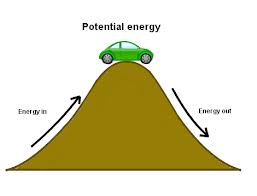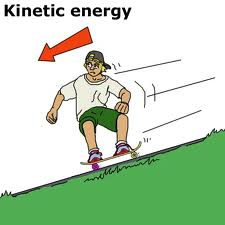# Difference between Kinetic Energy and Potential Energy

##### Key Difference: Potential energy is the energy in the stored form which is based on its position. On the other hand, kinetic energy can be referred to as the energy of an object that is based on its motion.Energy can be described as the ability to do a certain task. Energy can be broadly categorized into two types of energies - Potential and Kinetic Energy. Potential energy is the energy in the stored form; meaning that the potential for performing work is present. On the other hand, kinetic energy depicts the energy of motion.

Potential energy is possessed by a body in the stored form. It results by virtue of a body’s position or its configuration. Many forms of energy are grouped under the category of Potential Energy. Some kind of force is always associated with an object that acts in conjunction with the physical property of matter. The physical property of the matter could be its mass, charge, elasticity, temperature, etc.The energy of an object that is possessed by it because of its motion is known as Kinetic energy. This form of energy is connected to the speed of an object. It is important to mention that in many mechanisms, energy is transferred backwards and forwards between the states of Potential and Kinetic energies. For example, if a stone is thrown upwards, it starts with some kinetic energy. It moves upward and starts losing its kinetic energy. At some point it momentarily stops. This particular point reflects the maximum potential energy and zero kinetic energy of the object. The object starts falling down and the potential energy gets converted to kinetic energy.

Comparison between Potential Energy and Kinetic Energy:

 Potential Energy Kinetic Energy Definition Potential energy is due do position, composition or arrangement. Kinetic energy is energy due to motion. Formula mass of the object x acceleration of Gravity x Height of the object, PE = m x g x h 1/2 x mass of the object x speed of the object², KE = ½ x M x V². S.I. Unit Joule Joule Example Wound up spring of a toy, a stone on top of a hill, etc. A person who is walking, a pen falling from a table, etc. Types Gravitational, Elastic and Chemical Potential Energy. Rotational and Translational Kinetic Energy.

Image Courtesy: formulas.tutorvista.com, tooraps.vic.edu.au